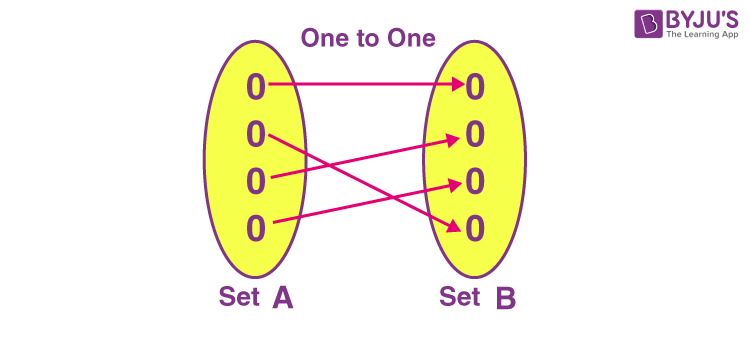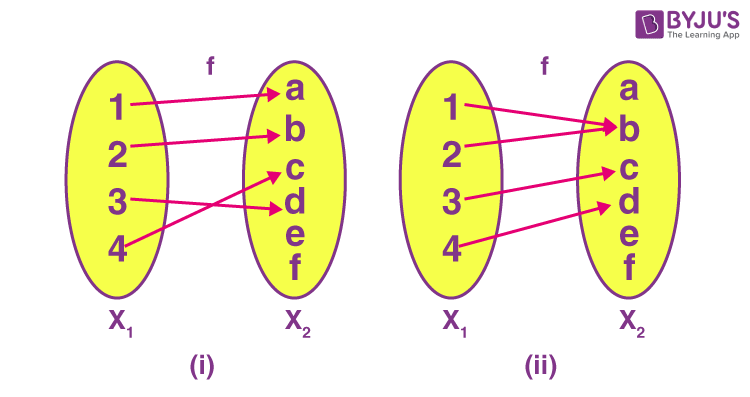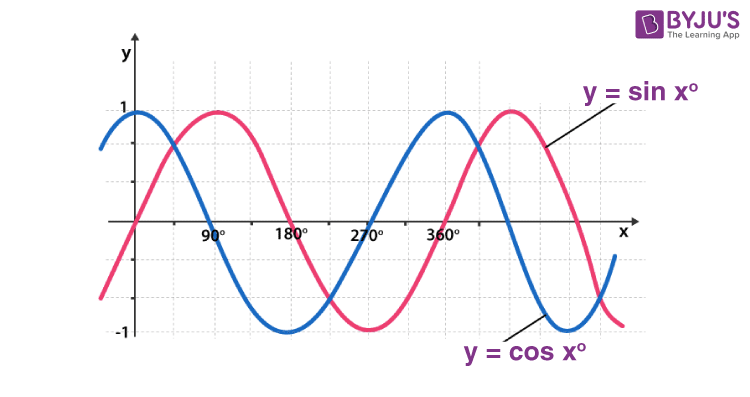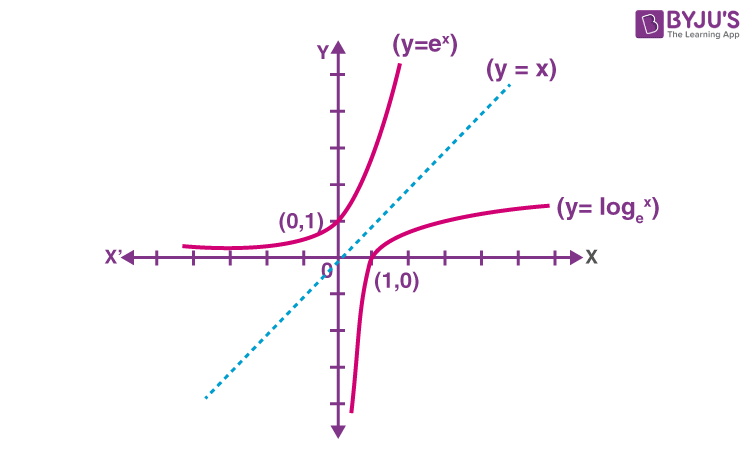# One to One Function

One to one function basically denotes the mapping of two sets. A function g is one-to-one if every element of the range of g corresponds to exactly one element of the domain of g. One-to-one is also written as 1-1. A function f() is a method, which relates elements/values of one variable to the elements/values of another variable, in such a way that the elements of the first variable identically determine the elements of the second variable. Also, learn about the onto function here.

With the help of examples, we are going to learn about this function in detail so that its concept could be easily understood. This concept is widely explained in Class 11 and Class 12 syllabus.

Apart from the one-to-one function, there are other sets of functions which denote the relation between sets, elements or identities. They are;

• Many to One function or Surjective function
• Onto Function or Bijective function

Also, we have other types of functions in Maths which you can learn here quickly, such as Identity function, Constant function, Polynomial function, etc. Let us now learn, a brief explanation with definition, its representation and example.

## Definition of One-to-One Functions

A function has many types, and one of the most common functions used is the one-to-one function or injective function. Also, we will be learning here the inverse of this function.One-to-One functions define that each element of one set, say Set (A) is mapped with a unique element of another set, say, Set (B).

Or

It could be defined as each element of Set A has a unique element on Set B.

Or

An injective function (injection) or one-to-one function is a function that maps distinct elements of its domain to distinct elements of its codomain.

In brief, let us consider ‘f’ is a function whose domain is set A. The function is said to be injective if for all x and y in A,

Whenever f(x)=f(y), then x=y

And equivalently, if x ≠ y, then f(x) ≠ f(y)

Formally, it is stated as, if f(x) = f(y)  implies x=y, then f is one-to-one mapped, or f is 1-1.

Similarly, if “f” is a function which is one to one, with domain A and range B, then the inverse of function f is given by;

f-1(y) = x ; if and only if f(x) = y

A function f : X → Y is said to be one to one (or injective function), if the images of distinct elements of X under f are distinct, i.e., for every x1 , x2 ∈ X, f(x1 ) = f(x2 ) implies x1  = x2 . Otherwise, it is called many to one function.

The below figure shows two functions, where (i) is the injective (one to one) function and (ii) is not an injective, i.e. many-one function.In Maths, an injective function or injection or one-one function is a function that comprises individuality that never maps discrete elements of its domain to the equivalent element of its codomain. We can say, every element of the codomain is the image of only one element of its domain.

## Examples

Examples of Injective Function

• The identity function X → X is always injective.
• If function f: R→ R, then f(x) = 2x is injective.
• If function f: R→ R, then f(x) = 2x+1 is injective.
• If function f: R→ R, then f(x) = x2 is not an injective function, because here if x = -1, then f(-1) = 1 = f(1). Hence, the element of codomain is not discrete here.
• If function f: R→ R, then f(x) = x/2 is injective.
• If function f: R→ R, then f(x) = x3 is injective.
• If function f: R→ R, then f(x) = 4x+5 is injective.

## One to One Graph – Horizontal Line Test

An injective function can be determined by the horizontal line test or geometric test.

1. If a horizontal line intersects the graph of the function, more than one time, then the function is not mapped as one-to-one.
2. If a horizontal line can intersect the graph of the function only a single time, then the function is mapped as one-to-one.

Consider a graph of the function (x) = sin x or cos x as given in the figure below.For this graph, when we draw a horizontal line, it intersects the graph of sin x or cos x at more than one point. Thus, these functions are not one-one functions.

The below figure shows the examples of one-one functions as these functions satisfy the horizontal line test.### Is Parabola a one to one function?

No, a parabola is not a 1-1 function. It can be proved by the horizontal line test.

A parabola is represented by the function f(x) = x2

Now, if we draw the horizontal lines, then it will intersect the parabola at two points in the graph. Hence, for each value of x, there will be two outputs for a single input.

## One to One Function Inverse

If f is a function defined as y = f(x), then the inverse function of f is x = f -1(y) i.e. f-1 defined from y to x. In the inverse function, the co-domain of f is the domain of f -1 and the domain of f is the co-domain of f -1.

Only one-to-one functions have its inverse since these functions have one to one correspondences, i.e. each element from the range corresponds to one and only one domain element.

Let a function f: A -> B is defined, then f is said to be invertible if there exists a function g: B -> A in such a way that if we operate f{g(x)} or g{f(x)} we get the starting point or value.

Let us understand with the help of an example,

Example:

Show that the function f : X -> Y, such that f(x)= 5x + 7,

For all x, y  ∈ N is invertible.

Solution:

Let y N -> y = f(x) = 5x + 7 for x N

x = (y-7)/5

If we define h : Y -> X by h(y) = (y-7)/ 5

Again h ∘ f(x) = h[ f(x) ] = h{ 5x + 7 } = 5(y-7) /  5 + 7 = x

And f ∘ h(y) = f [ h(y) ] = f( (y-7) / 5) = 5(y-7) /  5 + 7 = y

Hence f is an invertible function and h is the inverse of f.

## Properties of One-One Function

• If f and g are both one to one, then f ∘ g follows injectivity.
• If g ∘ f is one to one, then function f is one to one, but function g may not be.
• f: X → Y is one-one, if and only if, given any functions g, h : P → X whenever f ∘ g = f ∘ h, then g = h. In other words, one-one functions are exactly the monomorphisms in the category set of sets.
• If f: X → Y is one-one and P is a subset of X, then f-1 (f(A)) = P. Thus, P can be retrieved from its image f(P).
• If f: X → Y is one-one and P and Q are both subsets of X, then f(P ∩ Q) = f(P) ∩ f(Q).
• If both X and Y are limited with the same number of elements, then f: X → Y is one-one, if and only if f is surjective or onto function.

## Solved Problems

Example 1:

Let A = {1, 2, 3} and B = {a, b, c, d}. Which of the following is a one-to-one function?

1. {(1, c), (2, c)(2, c)}
2. {(1, a),(2, b),(3, c)}
3. {(1, b)(1, c)}

Explanation: Here, option number 2 satisfies the one-to-one condition, as elements of set B(range) are uniquely mapped with elements of set A(domain).

Example 2:

Show that f: R→ R defined as f(a) = 3a3 – 4 is one to one function?

Solution:

Let f ( a1 ) = f ( a2 ) for all a1 , a∈ R

so 3a13 – 4 = 3a23 – 4

a13 = a23

a13 – a23 = 0

(a1 – a2) (a1 + a1a2 + a22) = 0

a1 = a2 and (a12 + a1a2 + a22) = 0

(a12 + a1a2 + a22) = 0 is not considered because there are no real values of a1 and a2.

Therefore,  the given function f is one-one.

Students are advised to solve more of such example problems, to understand the concept of one-to-one mapping clearly. To learn more about various Maths concepts, register with BYJU’S. Also, download its app to get personalised learning videos.

## Frequently Asked Questions – FAQs

Q1

### What is one to one function?

One to one function or one to one mapping states that each element of one set, say Set (A) is mapped with a unique element of another set, say, Set (B), where A and B are two different sets. It is also written as 1-1. In terms of function, it is stated as if f(x) = f(y) implies x = y, then f is one to one.
Q2

### What is an injective function?

An injective function is nothing but one to one function, where each element of one set is mapped with each element of another set.
Q3

### Give examples of one to one function.

If f:R→ R is a function, then the examples of one to one are:
f(x) = 2x
f(x) = x3
f(x) = 3x+1
f(x) = x/3
Q4

### Which function is not said to be one to one?

If a horizontal line can intersect the graph of the function, more than one time, then the function is not mapped as one-to-one.
Q5

### What is onto function?

If for every element of B, there is at least one or more than one element matching with A, then the function is said to be onto function or surjective function.
Quiz on One to one function